An illustration showing a quadrilateral inscribed in a circle that is tangent to a line.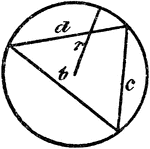Triangle Inscribed In A Circle

An illustration showing a triangle with sides b, c, and d inscribed in a circle with radius r.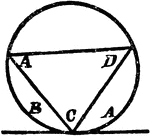Triangle Inscribed In A Circle

An illustration showing a triangle with angles A, C, and D inscribed in a circle which is tangent to…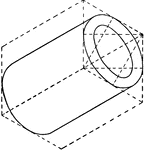Hollow Cylinder

Illustration of the isometric of a hollow cylinder.Hollow Cylinder

Illustration of a shaded section of a hollow cylinder viewed from the side.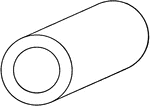Oblique View Of Hollow Cylinder

Illustration of an oblique view of a hollow cylinder.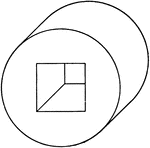Oblique View Of Hollow Cylinder

Illustration of an oblique view of a hollow cylinder. The portion removed from the center of the cylinder…Equilateral Triangle Inscribed In An Equilateral Triangle

Illustration used to prove that triangle EFD is equilateral given that triangle ABC is equilateral and…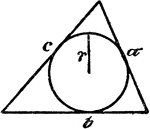Circle Inscribed In A Triangle

An illustration showing a circle with radius r inscribed in a triangle with sides a, b, and c.Circle Inscribed In A Triangle

An illustration showing a circle with sectors D and E inscribed in a triangle with angles, A, B, and…